• No products in the cart.

# 203.5.8 Neural Network Algorithm-Demo

##### Simple test case implementation of NN algorithm.

In the previous post we briefly discussed how the Neural Network Algorithm works. In this post we will implement the algorithm on a simple case.

### Neural network Algorithm-Demo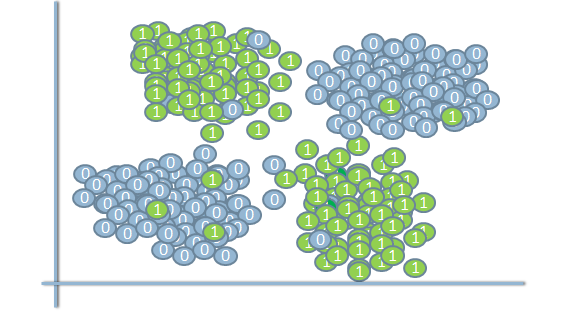Looks like a dataset that can’t be separated by using single linear decision boundary/perceptron

• Lets consider a similar but simple classification example
• XOR Gate Dataset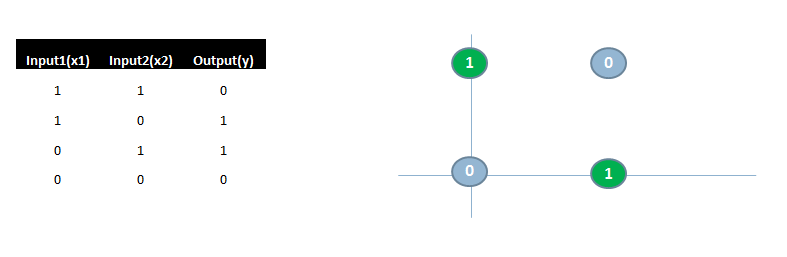#### Randomly Initialize Weights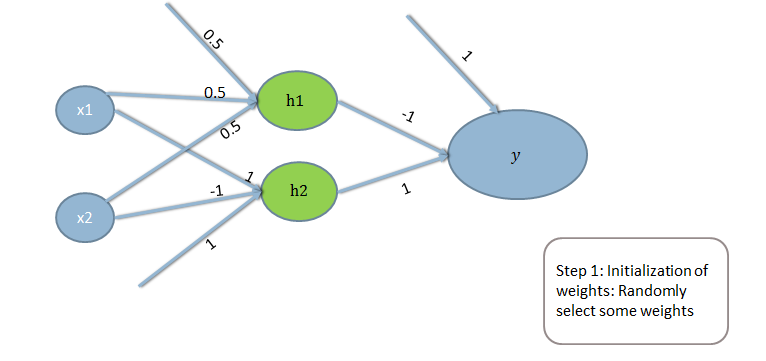#### Activation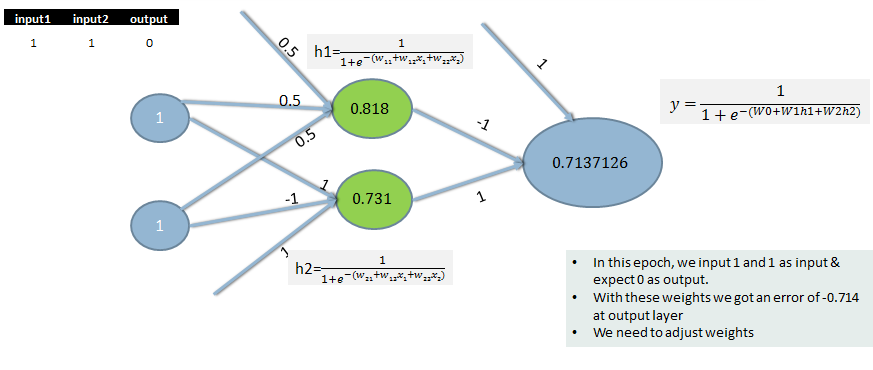#### Back-Propagate Errors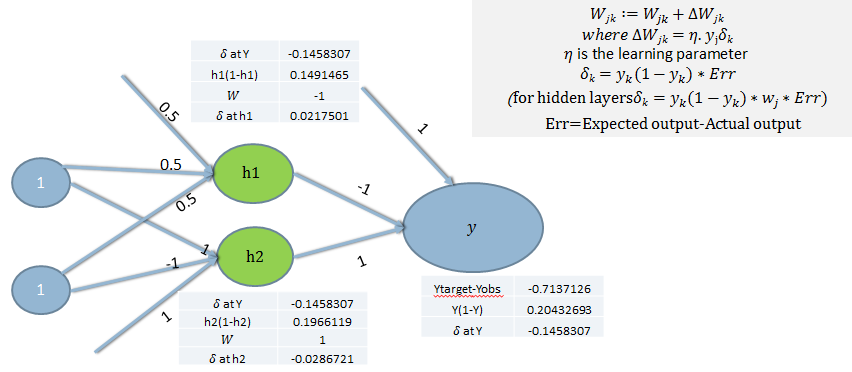#### Calculate Weight Corrections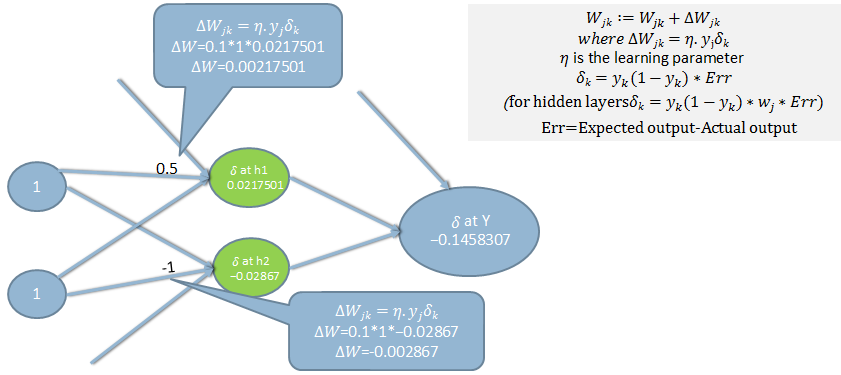#### Updated Weights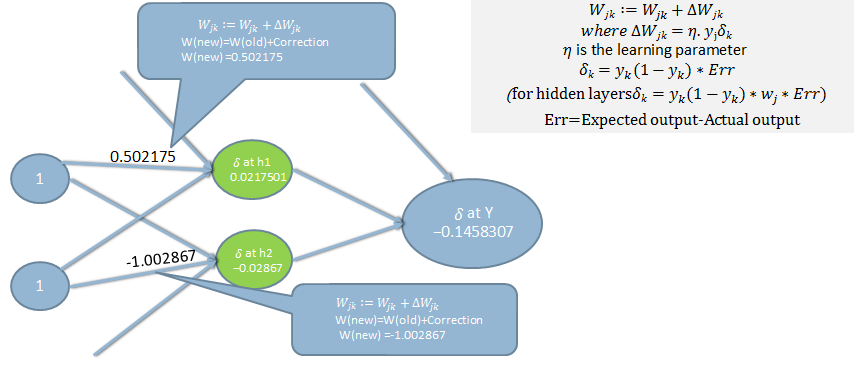#### Updated Weights contd…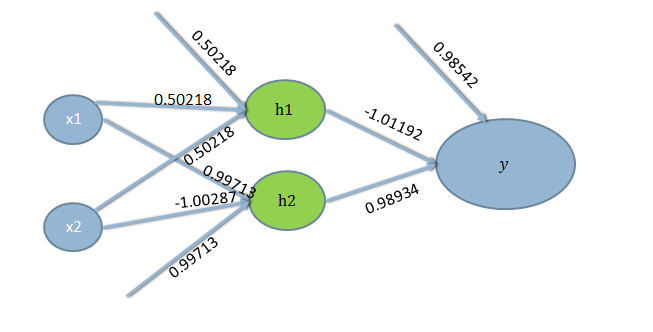#### Iterations and Stopping Criteria

• This iteration is just for one training example (1,1,0), this is just the first epoch.
• We repeat the same process of training and updating of weights for all the data points.
• We continue and update the weights until we see there is no significant change in the error or when the maximum permissible error criteria is met.
• By updating the weights in this method, we reduce the error slightly. When the error reaches the minimum point the iterations will be stopped and the weights will be considered as optimum for this training set.

XOR Gate final NN Model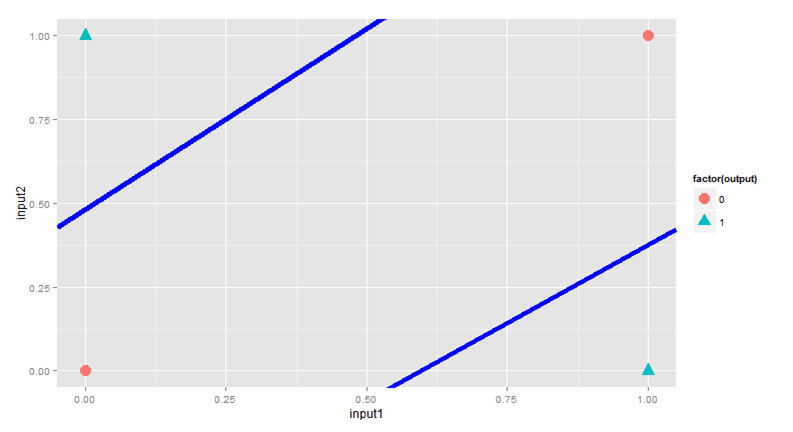The next post is about Building a Neural Network in R.
21st June 2017
0 responses on "203.5.8 Neural Network Algorithm-Demo"